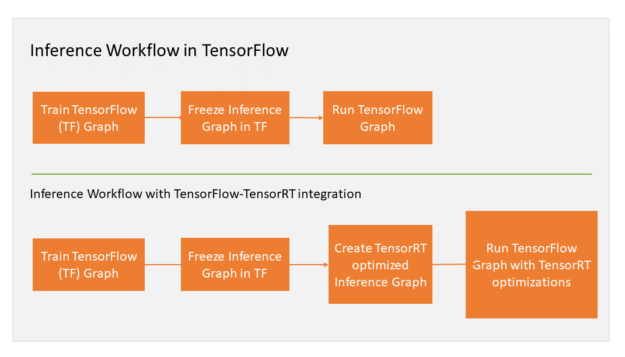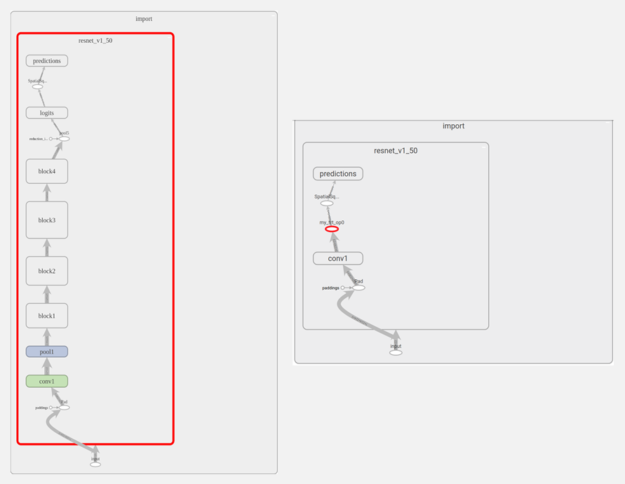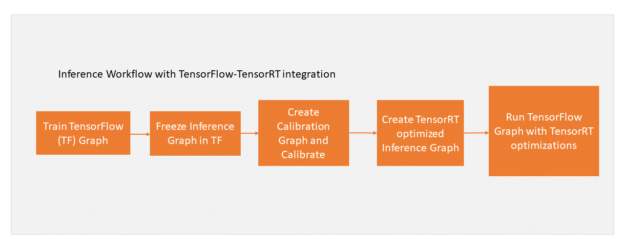# TensorRT Integration Speeds Up TensorFlow InferenceUpdate, May 9, 2018: TensorFlow v1.7 and above integrates with TensorRT 3.0.4. NVIDIA is working on supporting the integration for a wider set of configurations and versions. We’ll publish updates when these become available. Meanwhile, if you’re using `pip install tensorflow-gpu`, simply download TensorRT files for Ubuntu 14.04 not16.04, no matter what version of Ubuntu you’re running.

## Overview

NVIDIA announced the integration of our TensorRT inference optimization tool with TensorFlow. TensorRT integration will be available for use in the TensorFlow 1.7 branch. TensorFlow remains the most popular deep learning framework today while NVIDIA TensorRT speeds up deep learning inference through optimizations and high-performance runtimes for GPU-based platforms. We wish to give TensorFlow users the highest inference performance possible along with a near transparent workflow using TensorRT. The new integration provides a simple API which applies powerful FP16 and INT8 optimizations using TensorRT from within TensorFlow. TensorRT sped up TensorFlow inference by 8x for low latency runs of the ResNet-50 benchmark.

Let’s take a look at the workflow, with some examples to help you get started.

## Sub-Graph Optimizations within TensorFlow

TensorFlow integration with TensorRT optimizes and executes compatible sub-graphs, letting TensorFlow execute the remaining graph. While you can still use TensorFlow’s wide and flexible feature set, TensorRT will parse the model and apply optimizations to the portions of the graph wherever possible. Your TensorFlow program requires only a couple of new lines of code to facilitate the integration. Are you already using TensorRT with TensorFlow models? Then you know that applying TensorRT optimizations to TensorFlow models requires exporting the graph. You may need to manually import certain unsupported TensorFlow layers.

Let’s step through the workflow. After freezing the TensorFlow graph for inference, you ask TensorRT to optimize TensorFlow’s sub-graphs. TensorRT then replaces each supported subgraph with a TensorRT optimized node, producing a frozen graph that runs in TensorFlow for inference. Figure 1 illustrates the workflow.Figure 1. Workflow Diagram when using TensorRT within TensorFlow During inference.

TensorFlow executes the graph for all supported areas and calls TensorRT to execute TensorRT optimized nodes. As an example, assume your graph has 3 segments, A, B and C. Segment B is optimized by TensorRT and replaced by a single node. During inference, TensorFlow executes A, then calls TensorRT to execute B, and then TensorFlow executes C. From a user’s perspective, you continue to work in TensorFlow as earlier. Let’s walk through an example applying this workflow.

## New TensorFlow APIs

Add a couple of lines to your existing TensorFlow GPU code to apply TensorRT optimizations to the TensorFlow graph using the new TensorFlow APIs. This will:

1. Specify the fraction of GPU memory allowed for TensorFlow. TensorRT can use the remaining memory.
2. Let TensorRT analyze the TensorFlow graph, apply optimizations, and replace subgraphs with TensorRT nodes.

Use the new `per_process_gpu_memory_fraction` parameter of the `GPUOptions` function to specify the GPU memory fraction TensorRT can consume. This parameter should be set the first time the TensorFlow-TensorRT process starts. As an example, 0.67 would allocate 67% of GPU memory for TensorFlow, making the remaining 33% available for TensorRT engines.

`gpu_options = tf.GPUOptions(per_process_gpu_memory_fraction = number_between_0_and_1)`

You apply TensorRT optimizations to the frozen graph with the new `create_inference_graph` function. TensorRT then takes a frozen TensorFlow graph as input and returns an optimized graph with TensorRT nodes. See the example code snippet below:

```trt_graph = trt.create_inference_graph(
input_graph_def=frozen_graph_def,
outputs=output_node_name,
max_batch_size=batch_size,
max_workspace_size_bytes=workspace_size,
precision_mode=precision)```

Let’s look at the function’s parameters:

• `frozen_graph_def`:                    frozen TensorFlow graphout
• `put_node_name`:                           list of strings with names of output nodes e.g. “resnet_v1_50/predictions/Reshape_1”]
• `max_batch_size`:                         integer, size of input batch e.g. 16
• `max_workspace_size_bytes`:   integer, maximum GPU memory size available for TensorRT
• `precision_mode`:                         string, allowed values “FP32”, “FP16” or “INT8”

You should use the `per_process_gpu_memory_fraction` and `max_workspace_size_bytes` parameters together for best overall application performance. For example, set the `per_process_gpu_memory_fraction parameter` to ( 12 – 4 ) / 12 = 0.67 and the `max_workspace_size_bytes` parameter to 4000000000 for a 12GB GPU in order to allocate ~4GB for the TensorRT engines.

## Visualize Optimized Graph in TensorBoard

Let’s visualize the changes to the ResNet-50 node graph once TensorRT optimizations are applied in TensorBoard. As you can see in Figure 2, TensorRT optimizes almost the complete graph, replacing it with a single node titled “my_trt_op0” (highlighted in red). Depending on the layers and operations in your model, TensorRT nodes replace portions of your model due to optimizations.Figure 2. Left: ResNet-50 graph in TensorBoard. Right: After TensorRT optimizations and sub-graph replaced with TensorRT node.

Notice the node titled “conv1” below the TensorRT node. This is actually not a convolution layer, but rather a transpose operation from NHWC to NCHW which shows up as conv1.

## Use Tensor Cores Automatically

Using half-precision (also called FP16) arithmetic reduces memory usage of the neural network compared with FP32 or FP64. This allows deployment of larger networks, and takes less time than FP32 or FP64 transfers. The NVIDIA Volta Tensor Core provides a 4x4x4 matrix processing array which performs the operation D = A * B + C, where A, B, C, and D are 4×4 matrices as Fig. 3 shows. The matrix multiply inputs A and B are FP16 matrices, while the accumulation matrices C and D may be FP16 or FP32 matrices.

TensorRT automatically uses Tensor Cores in Volta GPUs for inference when using half-precision arithmetic. The peak performance of Tensor Cores on the NVIDIA Tesla V100 is about an order of magnitude (10x) faster than double precision (FP64) and about 4 times faster than single precision (FP32). Just use “FP16” as value for the `precision_mode` parameter in the `create_inference_graph` function to enable half precision, as shown below.

```trt_graph = trt.create_inference_graph(getNetwork(network_file_name), outputs,
max_batch_size=batch_size, max_workspace_size_bytes=workspace_size, precision_mode="FP16")```

Figure 4 shows ResNet-50 performing 8x faster under 7 ms latency with the TensorFlow-TensorRT integration using NVIDIA Volta Tensor Cores versus running TensorFlow only.

## Optimized INT8 Inference Performance

Performing inference using INT8 precision further improves computation speed and places lower requirements on bandwidth. The reduced dynamic range makes it challenging to represent weights and activations of neural networks. Table 1 illustrates dynamic range effects.

 Dynamic Range Minimum Positive Value FP32 -3.4×1038 ~ +3.4×1038 1.4 × 10-45 FP16 65504 ~ +65504 5.96 x 10-8 INT8 -128 ~ +127 1

Table 1: FP32 vs FP16 vs INT8 dynamic range

TensorRT provides capabilities to take models trained in single (FP32) and half (FP16) precision and convert them for deployment with INT8 quantizations while minimizing accuracy loss.

To convert models for deployment with INT8, you need to calibrate the trained FP32 model before applying TensorRT’s optimizations described in the earlier sections. The remaining workflow remains unchanged. Figure 5 shows the updated workflow.Figure 5. Updated TensorFlow workflow including INT8 calibrations prior to TensorRT optimizations

First use the `create_inference_graph function` with the `precision_mode parameter` set to “INT8” to calibrate the model. The output of this function is a frozen TensorFlow graph ready for calibration.

```trt_graph = trt.create_inference_graph(getNetwork(network_file_name), outputs,
max_batch_size=batch_size, max_workspace_size_bytes=workspace_size, precision_mode="INT8")```

Next, execute the calibration graph with calibration data. TensorRT uses the distribution of node data to quantize the weights for the nodes. It is important to use calibration data that closely reflects the distribution of the problem dataset in production. We suggest checking for error accumulation during inference when first using models calibrated with INT8.

After executing the graph on calibration data, apply TensorRT optimizations to the calibration graph with the `calib_graph_to_infer_graph` function. This function also replaces the TensorFlow subgraph with a TensorRT node optimized for INT8. The output of the function is a frozen TensorFlow graph that can be used for inference as usual.

`trt_graph=trt.calib_graph_to_infer_graph(calibGraph)`

And that’s it! These two commands enable INT8 precision inference with your TensorFlow model.

All code required to run these examples can be found here.

## TensorRT Integration Availability

We continue to work closely with the TensorFlow team to enhance integration for this exciting release. We expect the new solution to ensure the highest performance possible when using NVIDIA GPUs while maintaining the ease and flexibility of TensorFlow. Developers will automatically benefit from updates as TensorRT supports more networks, without any changes to existing code.

You can find detailed installation instructions on how to get started at the TensorFlow site.

The integration will also be available in the NVIDIA GPU Cloud (NGC) TensorFlow container. We believe you’ll see substantial benefits to integrating TensorRT with TensorFlow when using GPUs for inference. The TensorRT page offers more information on TensorRT as well as links to further articles and documentation. NVIDIA strives to constantly improve its technologies and products, so please let us know what you think by leaving a comment below.